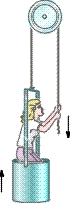# Some trouble with my force calculations

big_d

## Homework Statement

I have 2 problems I'm having trouble with right now.(actually more but I'll ask 2 and try to figure out the others for now.

Two blocks are in contact on a frictionless table. A horizontal force F is applied to M2, as shown. If M1 = 1.06 kg, M2 = 3.38 kg, and F = 4.75 N, find the magnitude of the contact force between the two blocks.
I calculated the acceleration of the entire system to be 1.069m/s^2 but don't know where to go from there.

A window washer pulls herself upward using the bucket-pulley apparatus shown in figure below.

How hard must she pull downward to raise herself slowly at constant speed? The mass of the person plus the bucket is 61.5kg.

Correct, computer gets: 3.01e+02 N

If she increases this force by 10.3 percent, what will her acceleration be?
I answered the first part, and for the second part have determined that if she increases her force ten percent to ~331N, that the acceleration upward would be 662N-601N/mass, but this is incorrect.

F=ma?

Last edited:

## Answers and Replies

big_d
I keep doing the second one like this, and keep getting .98m/s/s

301x1.1=331.1x2=661-601=60.1/61.5kg=.98m/s/s which is not right

Last edited:
Staff Emeritus
Gold Member
Draw a free body diagram of each block separately and analyse the forces acting on it.

big_d
Draw a free body diagram of each block separately and analyse the forces acting on it.

OK I just did so and got the 4.75N initial force acting on M2, 3.6132N force acting due to acceleration, and on m1 I got 1.13314N in the same direction, so would I do 4.75+3.6132-1.13314? Or does the force from the push carry over to M1 as well?

Staff Emeritus
Gold Member
I'm not sure what you mean with that but 3.613N is the answer. No further action needed.

big_d
I'm not sure what you mean with that but 3.613N is the answer. No further action needed.
I entered that and it said it was wrong? Weird

Staff Emeritus
Gold Member
I entered that and it said it was wrong? Weird

Sorry, it was very close to what I got as an answer but not exactly the same. Try calculating again.

big_d
Sorry, it was very close to what I got as an answer but not exactly the same. Try calculating again.

I tried, all my tries are up so I missed it. Can anyone help on the other one?

Staff Emeritus
Gold Member
I'm surprised they were so strict, it was only 0.003 off.

Last edited:
big_d
Ya thats weird.big_d
can you guys see that picture?

Homework Helper
Hi big_d,

OK I just did so and got the 4.75N initial force acting on M2, 3.6132N force acting due to acceleration, and on m1 I got 1.13314N in the same direction, so would I do 4.75+3.6132-1.13314? Or does the force from the push carry over to M1 as well?

I believe the answer for the first problem would be 1.13 N. The acceleration of the entire system is 1.07 m/s^2, and the contact force is the only horizontal force acting on m1 (1.06 kg), so using F=ma gives 1.13 N.

If you want to get the same thing from m2, you would say that the force 4.75 is pushing in the direction of acceleration, the unknown contact force is pushing backwards, and the acceleration is 1.07 m/s^2, so using

(net force) = m a

gives

(4.75) - (contact force) = (3.38) (1.07) ---> contact force = 1.13 N

Last edited:
Homework Helper
I keep doing the second one like this, and keep getting .98m/s/s

301x1.1=331.1x2=661-601=60.1/61.5kg=.98m/s/s which is not right

I think this is the right procedure. However, when you don't round off the intermediate values, I get a value that is about 3 percet different from yours. Is that enough for the software to say the answer is wrong?

Staff Emeritus